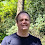### Solution: What is this broken code doing

Following my earlier blog, here is my solutions to these puzzles.

## Loop every second value

```List list = new ArrayList<>();
for (int i = 0; i < 10; i++) list.add(i);

for (int i = 0; i < list.size(); i++) {
System.out.println(list.get(i));
list.remove(i);
}
```

When you call remove(i) it shifts all the elements down one AND i++ shifts the index up one. This means, you see every second value.

A work around is as follows.

```while(!list.isEmpty)
System.out.println(list.remove(0));
```

## Endless loop

This prints all the characters which have value
```for(char ch = Character.MIN_VALUE; ch<= Character.MAX_VALUE; ch++) {
int i = Character.getNumericValue(ch);
if (i >= 0)
System.out.println("char "+ch + ' ' + (int) ch+" = "+i);
}
```
As many realised, this doesn't stop because a char is always less than or equals to the maximum value. (Which is why its called the maximum ;)

Some suggested avoiding this difficult value and have the loop iterate one less. However, another approach is to use a do/while loop which most developers don't use much AFAIK.

```char ch = Character.MIN_VALUE;
do {
int i = Character.getNumericValue(ch);
if (i < 0) continue;
System.out.println("char "+ch + ' ' + (int) ch+" = "+i);
} while(ch++ < Character.MAX_VALUE)
```
The difference is that the check is at the end of the loop instead of the start. This means its stops after ch = MAX_VALUE instead of before it iterates with this value.

## What am I waiting for?

This "program" deadlocks. The main method is optional ;)
```class Main {
static int value;

static {
@Override
public void run() {
value = 1; // attempts to set Main.value
}
};
t.start();
try {
t.join();
} catch (InterruptedException e) {
e.printStackTrace();
}
System.out.println("Released");
}
}
```
The problem here is that class initialization is single threaded and implicitly locked. This means another thread cannot use or access the class or its fields or method while another thread is initialising it. Using pseudo-Java it looks like this.
```class Main {
static int value;

static {
// synchronized(Main.class) {
@Override
public void run() {
// synchronized(Main.class) {
value = 1; // attempts to set Main.value
// }
}
};
t.start();
try {
t.join();
} catch (InterruptedException e) {
e.printStackTrace();
}
System.out.println("Released");
// }
}
}
```

1."The problem here is that class initialization is single threaded and implicitly locked"

This is what I wanted to know.

I'm learning a lot with your blog.

Thank you very much.

2.@Javier Thank you for the feedback. I hope people will learn something from my blogs. ;)